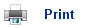# Acid-Base Reactions

• An acid is a substance that turns litmus paper red, reacts with carbonates to give CO2 and is neutralized by bases.
• Acids have the ability to transfer H+ ions to something else.
• The substance which accepts the ions is called a base.

For example:

HCl (g) + NH3(g)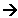NH4+Cl- (s)

• In this reaction the HCl has transferred H+ ions and the NH3 has accepted the ions. The HCl is the acid, and the NH3 is the base.
• In the above reaction, the oxidation states of the elements remain unchanged.
• Since H+ ions only consists of only one proton, we can refer to acids as proton donors, and bases as proton acceptors.
• The theory of H+ transfer is known as the BrŘnstead-Lowry theory of acids and bases.

## Acids and Bases in Solutions

• Water consists mainly of H2O molecules, and hydrogen chloride is a gas that contains HCl molecules.
• When these two substances mix, the solution (Hydrogen Chloride in water) easily conducts electricity, so it must contain H+ ions.
• The reaction between the hydrogen chloride molecules and the water molecules produces these ions: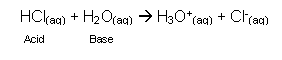• In this reaction, the hydrochloric acid is the acid (it is the proton donor) and the water is the base (proton acceptor).
• The H3O+ ion is called the oxonium ion and it is present in every acidic solution. The acid donates H+ to H2O forming H3O+.
• The H3O+ can now act as an acid; it can donate an H+ ion to a base.
• The properties of an acid are the same as the properties of the H3O+ ion.
• The H3O+ ion is often shortened to just H+

HCl (aq)H+ (aq) + Cl- (aq)

• An acid dissolves in water to form H+ ions.
• An Alkali dissolves in water to form OH- ions.
• Some alkalis such as sodium hydroxide contain OH- ions, whereas others, such as ammonia, form OH- ions when they react with water.

NH3(aq) + H2O (l)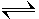NH4+ (aq) + OH-

## Acid-base pairs

• After an acid has donated its H+ ion, it can then accept it again:

CH3COOH (aq)CH3COO- (aq) + H+ (aq)

• When ethanoic acid acts as an acid, it donates its H+ ion (above).
• If a stronger acid is then added to the solution containing the ethanoic ions (CH3COO-), then they can act as a base, and accept H+ ions:

CH3COO- (aq) + H+(aq)CH3COOH(aq)

• When the acid ion acts as a base, it is called a conjugate base. In the above reaction, the ethanoic ion is the conjugate base of ethanoic acid.
• Every acid has a conjugate base, and every base has a conjugate acid.
• We refer to them as conjugate acid-base pairs.
• The general formula for a conjugate acid-base pair is summarised below.
HA(aq)H + A-

## Strengths of acids and bases

• Acids can have different strengths, some are powerful H+ donors, whereas others aren’t quite as strong.
• Powerful H+ donors are called strong acids.
• Weak H+ donors are called weak acids.
• A strong acid has a weak conjugate base, and a strong base has a weak conjugate acid.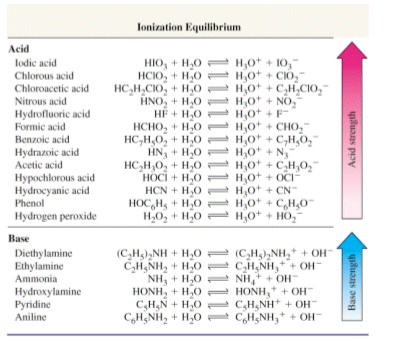## Indicators

• Most acid-base indicators are weak acids. The conjugate acid and base forms have different colours.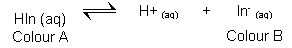• When acid (H3O+) is added the position of equilibrium shifts to the left, producing colour A.
• When Alkali (OH-) is added the psoition of equilibrium shifts to the right, producing colour B.
• Litmus is an example of this, showing red in its base state when an acid is present, and blue in its acid state, when a base is added.

## Useful books for revision:

Revise AS Chemistry for Salters (Written by experienced examiners and teachers of Salter's chemistry)Revise AS Chemistry for Salters (OCR) (Salters Advanced Chemistry)Home